# Introduction

Accurately comparing the performance of models with different hyperparameters and design choices is a core part of machine learning. Validation is a general techinique used to compare design and training choices during model development. This notebook investigates the tradeoffs from different ways of performing validation. Specifically: 1) the relationship between training, validation, and testing accuracy, 2) the effects of validation split size on the variance in performance metrics and model accuracy, and 3) how cross validation reduces variance in validation metrics for small datasets.

# Background

Developing a model requires numerous design decisions such as sizes of intermediate layers and choice of activation function. Additionally there are parameters controlling the training process such as learning rate, regularization strength, weight initialization, dropout probability, etc. These are called hyperparameters because they are parameters controlling the process of creating a model. In contrast non-hyper parameters are the numerical values that represent the model that the training process learns. These parameters are usually called model weights. Selecting hyperparameters requires spectial care and is decribed below.

We want the models we train to perform well both on the data we already have as well as on the data the model may see in the future. We collect a representative dataset for the problem and partition it into training, validation, and test data. The training data is used by the learning algorithm to select model weights and the test data is used to estimate how the model will perform on future data (e.g. when the model is deployed).

Test data must never be used to select hyperparameter values. Test data must only be used, in order for performance estimates of the final model about to be deployed to production to be accurate. If multiple models are evaluated on the test data, then the test data has contaminated the model developent process and the performance on the "test" data is not longer representative of generalization ability because the data has been seen before and may have influence decisions in the training process. Additionally the training and test data must be representative of the data we expect the model to see in the wild.

Training data for learning model weights must not be used for selecting hyperparameter values. The training process can produce models memorizing their training data. They have high accuracy on the training data, but perform poorly on new data they have not memorized. This is called overfitting. Because of overfitting, training performance is not a reliable indicator of how well a model will generalize. If the training accuracy of a model trained with one hyperparameter value is higher than the accuracy of another, it could be because the model overfit rather than the hyperparameter being better.

The purpose of validation data is to accurately compare different hyperparameter values. We train a model for each hyperparameter value using the training dataset and evaluate the model performance using the validation set. Because the validation set is not used to learn model weights, it provides a more reliable estimate of how the model will generalize. Because the validation set is only a sample of the entire dataset, some models may get luck and perform better than other models on the validation set even when both perform the same on the dataset as a whole. This means that it is possible to overfit to the validation set by trying out too many hyperparameters. Additionally, comparing training performance and validation performance during training provides an indication when a model is starting to overfit its data. When a gap develops between training and validation accuracy, the model is overfitting.

# Dataset and Model

These experiments use a synthetic dataset for a binary classification problem. Below is the code for generating the dataset, training a classifier, and evaluating the classifier Binary Cross-Entropy loss and prediction accuracy as performance metrics. Accuracy is the percent of samples where the model assigns >50% probability to the correct class. Binary Cross-Entry loss measures the difference between the predicted probability distribution and the actual 0-1 distribution of what the class label is.

```import matplotlib.pyplot as plt
import matplotlib.ticker as mtick
import pandas as pd
import torch
import torch.nn as nn
import torch.optim as optim
from sklearn.datasets import make_classification
from sklearn.model_selection import train_test_split```
```def make_dataset(n_samples=1000):
X, y = make_classification(n_samples=n_samples, n_features=10, n_classes=2, flip_y=0.01)
X_train, X_test, y_train, y_test = train_test_split(X, y, test_size=0.2)
```X_train_full, y_train_full, X_test, y_test = make_dataset()
X_train, X_val, y_train, y_val = train_test_split(X_train_full, y_train_full,
test_size=0.2)```
```def train_model(X_train, y_train, X_val=None, y_val=None,
epochs=256):
model = nn.Sequential(
nn.Linear(10, 64),
nn.ReLU(),
nn.Linear(64, 32),
nn.ReLU(),
nn.Linear(32, 1),
nn.Sigmoid(),
nn.Flatten(start_dim=0))
criterion = nn.BCELoss()

def evaluate_model(X, y):
output = model(X)
loss = criterion(output, y)
predicted = output > 0.5
accuracy = (predicted == y).float().mean()
return loss, accuracy

training_history_rows = []
training_history_column_names = ['Training Loss', 'Training Accuracy']
if X_val is not None:
training_history_column_names.extend(
['Validation Loss', 'Validation Accuracy'])
for epoch in range(epochs):
training_loss, training_accuracy = evaluate_model(X_train, y_train)
metrics = [training_loss.detach(), training_accuracy]
if X_val is not None:
validation_loss, validation_accuracy = evaluate_model(X_val, y_val)
metrics.extend([validation_loss, validation_accuracy])
training_history_rows.append(map(float, metrics))
training_loss.backward()
optimizer.step()
training_history = pd.DataFrame(dict(zip(training_history_column_names,
zip(*training_history_rows))))
return model, training_history

def evaluate_model(model, X, y, criterion=nn.BCELoss()):
output = model(X)
loss = criterion(output, y)
predicted = output > 0.5
accuracy = (predicted == y).float().mean()
return float(loss), float(accuracy)```

# Training and Validation Loss During Training

Below we train a classifier for 1000 epochs and plot the loss and accuracy for the training and validation sets at each epoch. At first both the training and validation losses decrease while the validation and training accuracies increase. At some point the model starts overfitting and the training and validation accuracies diverge with the training accuracy being higher than the validation accuracy. The reason the training accuracy is higher is the model has memorized part of the training set but cannot memorize the validation set because it does have access to it. As the model overfits more and more, the validation loss starts steadily increasing while the training loss continues to decrease.

```def train_vs_validation():
model, training_history = train_model(X_train, y_train, X_val, y_val,
epochs=1000)

epochs = torch.arange(1, len(training_history) + 1)
colors = ['red', 'blue', 'orange', 'green']
fig, ax1 = plt.subplots()
ax1.plot(epochs, training_history['Training Loss'],
label='Training Loss', color=colors)
ax1.plot(epochs, training_history['Validation Loss'],
label='Validation Loss', color=colors)
ax1.set_xlabel('Epoch')
ax1.set_ylabel('Loss')
ax2 = ax1.twinx()
ax2.plot(epochs, training_history['Training Accuracy'],
label='Training Accuracy', color=colors)
ax2.plot(epochs, training_history['Validation Accuracy'],
label='Validation Accuracy', color=colors)
ax2.set_ylabel('Accuracy')
fig.suptitle('Training vs Validation Accuracy')
fig.legend()
train_vs_validation()```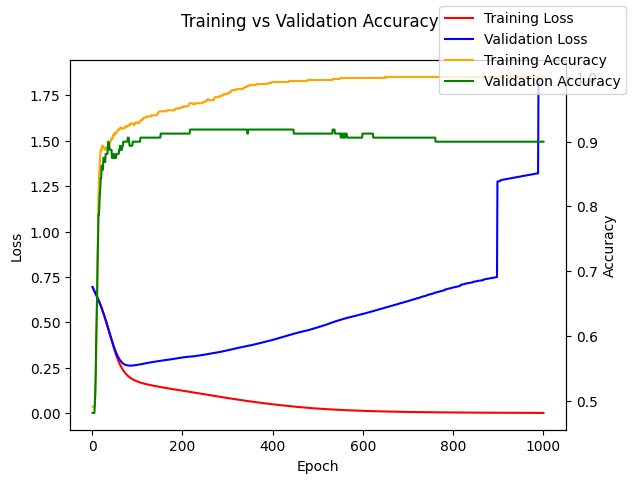# Methodology

The following experiments use a dummy hyperparameter that does not have any effect on the model to isolate the effects of hyperparameter selection techniques from the actual hyperparameters themselves. Differences is performance from one model to another are from the randomness in the training process (e.g. weight initialization) or the subsets of data used for training and validation (e.g. 20% train validation split vs 30%).

# Correlation Between Training, Validation, and Test Performance

This section looks at the correlation between training, validation, and test performance.

When a model is underfitting, the training, validation, and test accuracies are all positively correlated. This is because training accuracy increases by better fitting the general distribution of the dataset. As the model stops underfitting the accuraries become less correlated. In the first plot we can tell the models are not overfitting because the ranges of accuracies are similar across each of the sets.

When a model is overfitting there is a negative correlation between training and test accuracy. We can tell the models are overfitting in the later plots because the range of accuracies for the training set is higher than the range of values for the training and validation sets. Increasing training accuracy by additional overfitting hurts generalization performance which causes the negative correlation with both the testing and validation sets. There is still a positive correlation between validation accuracy and testing accuracy even when the model is overfitting. Additionally the range of accuracy values for the validation and testing sets are still similar. This is why validation accuracy is a better metric than training accuracy for comparing hyperparameters.

```def train_and_evaluate(epochs, X_train=X_train, y_train=y_train,
X_val=X_val, y_val=y_val, X_test=X_test, y_test=y_test):
model, training_history = train_model(X_train, y_train, epochs=epochs)
training_loss, training_accuracy = training_history.iloc[-1]
validation_loss, validation_accuracy = evaluate_model(model, X_val, y_val)
testing_loss, testing_accuracy = evaluate_model(model, X_test, y_test)
return {
'Training Accuracy': training_accuracy,
'Training Loss': training_loss,
'Validation Accuracy': validation_accuracy,
'Validation Loss': validation_loss,
'Testing Accuracy': testing_accuracy,
'Testing Loss': testing_loss,
}

def compare_training_vs_validation_vs_test(title, epochs):
metrics = pd.DataFrame([train_and_evaluate(epochs) for
meaningless_hyperparamter_value in torch.arange(100)])

def compare(metric_a, metric_b, ax):
correlation = metrics[metric_a].corr(metrics[metric_b])
metrics.plot.scatter(metric_b, metric_a, ax=ax)
ax.set_xlabel('%s\n(correlation %0.2f)' % (metric_b, correlation))

fig, axs = plt.subplots(2, 3, figsize=(8,8))

compare('Testing Accuracy', 'Validation Accuracy', axs[0, 0])
compare('Testing Accuracy', 'Training Accuracy', axs[0, 1])
compare('Validation Accuracy', 'Training Accuracy', axs[0, 2])

compare('Testing Loss', 'Validation Loss', axs[1, 0])
compare('Testing Loss', 'Training Loss', axs[1, 1])
compare('Validation Loss', 'Training Loss', axs[1, 2])

fig.suptitle(title)
fig.tight_layout()
plt.show()

compare_training_vs_validation_vs_test('Underfitting', epochs=25)
compare_training_vs_validation_vs_test('Crossover', epochs=150)
compare_training_vs_validation_vs_test('Overfitting', epochs=500)
# TODO: make video one frame per epoch showing the correlation plots```
```/usr/local/lib/python3.9/dist-packages/pandas/plotting/_matplotlib/core.py:1114: UserWarning: No data for colormapping provided via 'c'. Parameters 'cmap' will be ignored
scatter = ax.scatter(
```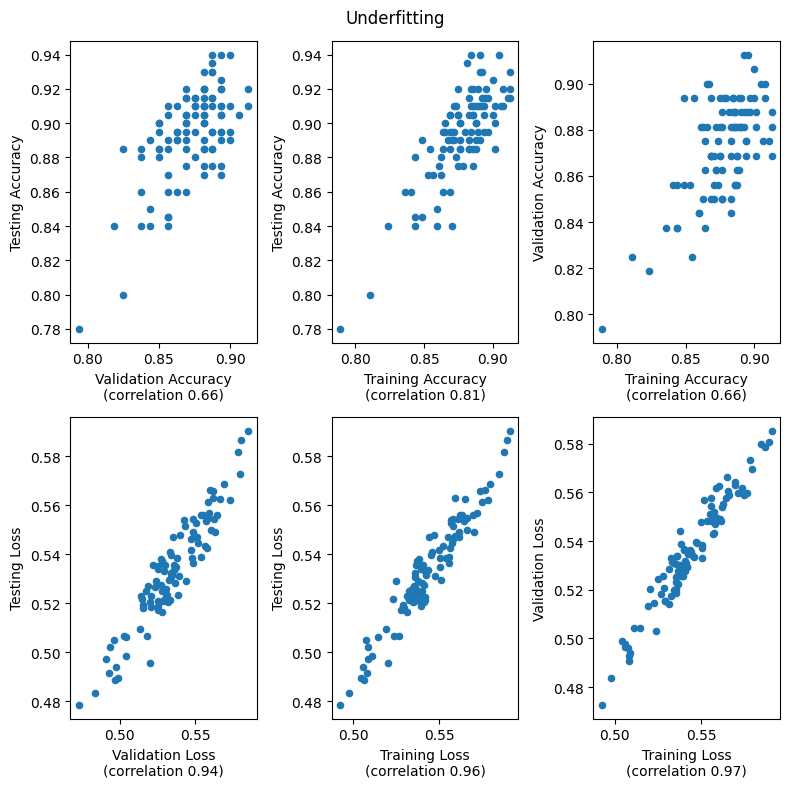```/usr/local/lib/python3.9/dist-packages/pandas/plotting/_matplotlib/core.py:1114: UserWarning: No data for colormapping provided via 'c'. Parameters 'cmap' will be ignored
scatter = ax.scatter(
```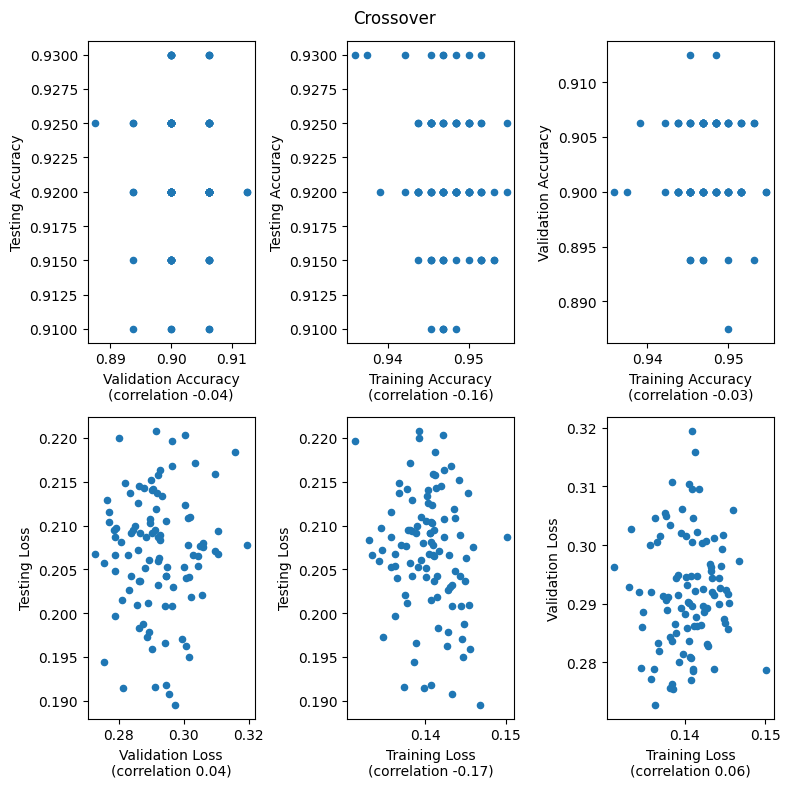```/usr/local/lib/python3.9/dist-packages/pandas/plotting/_matplotlib/core.py:1114: UserWarning: No data for colormapping provided via 'c'. Parameters 'cmap' will be ignored
scatter = ax.scatter(
```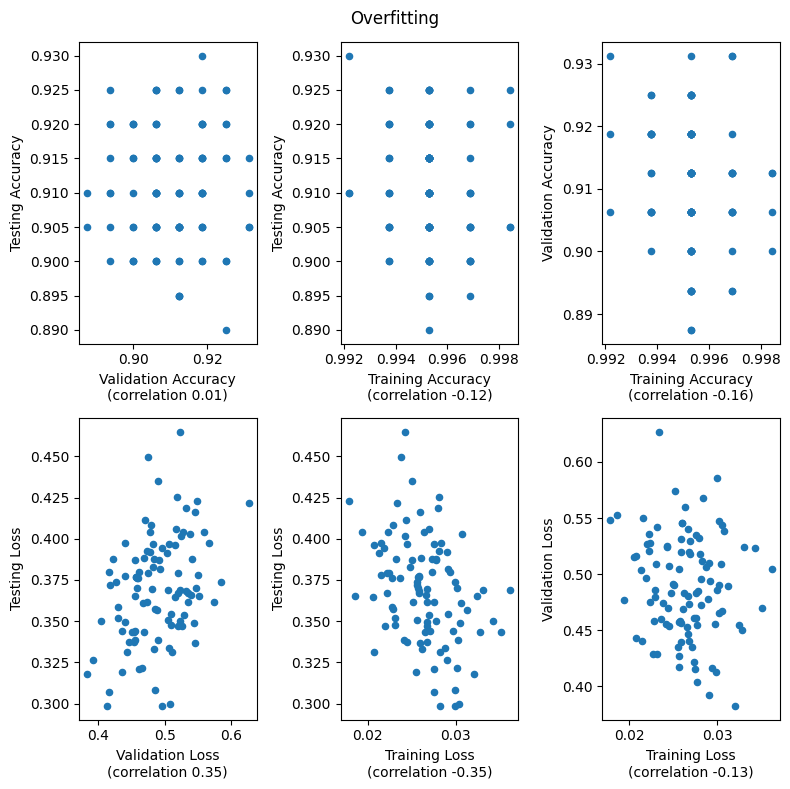# Validation Set Size

The smaller the validation set, the noiser the validation metrics. In the extreme case of a single validation datapoint, the validation accuracy with either be 0 or 1. Using more of the data for validation reduces the noise in the performance metrics because of the central limit theorem. Increasing the validation set size decreases the amount of data the model is trained on. Using too much of the data for validation at some point hurts model performance. Below in the extreme case of using 99% of the data for validation, model performance drops to 50% (ie random guessing).

```def compare_validation_split_sizes(epochs=100):
num_samples = 10
split_sizes = torch.arange(0.01, 1, 0.01).repeat(num_samples)
validation_accuracies = []
for size in split_sizes:
X_train, X_val, y_train, y_val = train_test_split(X_train_full, y_train_full,
test_size=size)
model, training_history = train_model(X_train, y_train, epochs=epochs)
_, validation_accuracy = evaluate_model(model, X_val, y_val)
validation_accuracies.append(validation_accuracy)

fig, ax = plt.subplots()
ax.scatter(split_sizes, validation_accuracies)
ax.set_xlabel('Validation Split Size')
ax.xaxis.set_major_formatter(mtick.PercentFormatter())
ax.set_ylabel('Accuracy')
fig.suptitle('Validation Accuracy vs Split Size')
compare_validation_split_sizes()```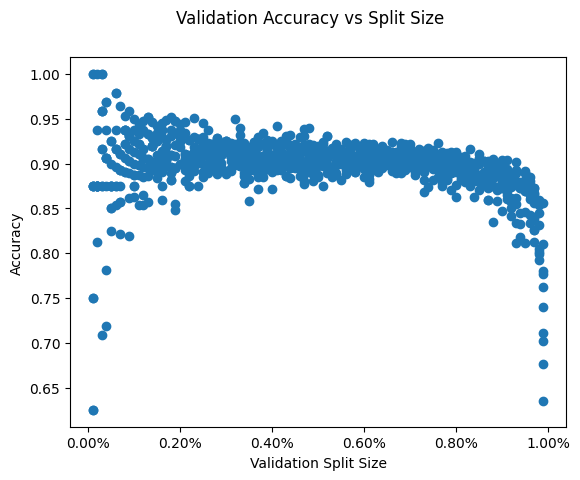# Cross Validation

Cross validation is a technique for reducing noise in validation set metrics for small datasets. The smaller the dataset, the smaller the validation set and the noisier the validation accuracy and loss will be. In the plots below the validation accuracies vary 5-10 percentage points for the small dataset vs 2 percentage points for the larger dataset. Cross validation splits the dataset into k folds where k is a hyperparameter (typically 2, 3, or 5). For the ith fold, cross validation trains a model on all of the dataset except for the ith fold and computes validation performance metrics using the ith fold. Cross validation reduces noise by averaging the validation performance from the k models and because every piece of the dataset contributes to the average.

For small datasets increasing the number of folds decreases the noise in the validation metrics. For larger datasets cross validation has limited usefullness. The validation metrics are not that noisy already and cross validation cannot reduce the noise by much more. k-fold cross validation requires O(k) times more computation compared to a single train-validation split because each piece of data is used for training k - 1 models. For small datasets this additional cost is worth the increased percision, however for larger datasets the cost grows prohibitive.

```def cross_validation_evaluation(num_folds, X_train_full, y_train_full):
fold_assignment = torch.arange(len(X_train_full)) % num_folds
accuracies = []
losses = []
for fold in range(num_folds):
X_val = X_train_full[fold_assignment == fold]
y_val = y_train_full[fold_assignment == fold]
X_train = X_train_full[fold_assignment != fold]
y_train = y_train_full[fold_assignment != fold]

model, _ = train_model(X_train, y_train, epochs=50)
loss, accuracy = evaluate_model(model, X_val, y_val)
losses.append(loss)
accuracies.append(accuracy)

def compare_num_folds(title, dataset_size=100):
X_train_full, y_train_full, X_test, y_test = make_dataset(dataset_size)

num_samples = 10
num_folds_range = torch.arange(2, 11).repeat(num_samples)
losses, accuracies = zip(*[
cross_validation_evaluation(num_folds, X_train_full, y_train_full) for
num_folds in num_folds_range])

fig, (ax1, ax2) = plt.subplots(2)
ax1.scatter(num_folds_range, accuracies)
ax1.set_xlabel('Num Folds')
ax1.set_ylabel('Cross Validation Accuracy')
ax1.title.set_text('Cross Validation Accuracy vs Num Folds')

ax2.scatter(num_folds_range, losses)
ax2.set_xlabel('Num Folds')
ax2.set_ylabel('Cross Validation Loss')
ax2.title.set_text('Cross Validation Loss vs Num Folds')
fig.suptitle(title)
fig.tight_layout()

compare_num_folds('Small Dataset', dataset_size=100)
compare_num_folds('Large Dataset', dataset_size=1000)```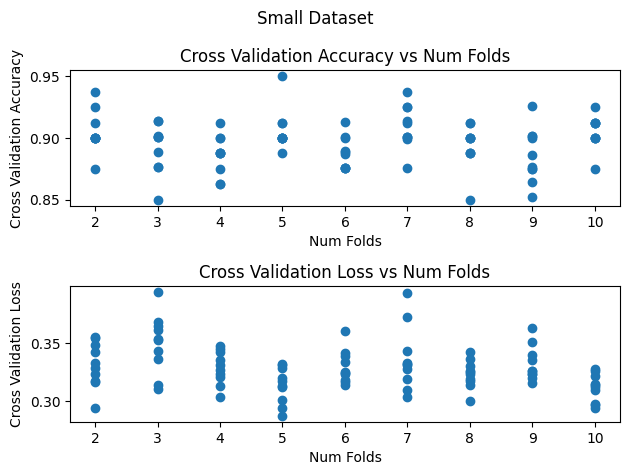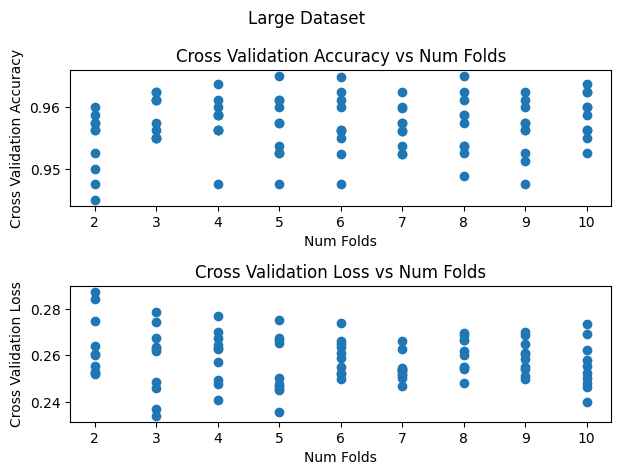# Overfitting the Validation Set

It is possible to overfit to the validation dataset by trying too many hyperparameter values. In the experiment below we train 10 a sequences of 1000 different models and keep track of the models with the best validation accuracy so far. In the plot below the validation accuracy of the best models monotonically increases and the validation loss generally decreases with the "better" models. In contrast the test accuracy for some of the selected models decreases even with when the validation accuracy increases.

```def overfitting_validation_set(epochs):
num_searches = 10
def search():
'''
Performs a hyperparamter search and returns a DataFrame where the ith row
has the metrics for model with the best validation accuracy from the first
i + 1 models.
'''
best_validation_accuracy = 0
best_metrics = None
best_metrics_vs_time = []
for meaningless_hyperparamter_value in torch.arange(50):
metrics = train_and_evaluate(epochs)
if metrics['Validation Accuracy'] > best_validation_accuracy:
best_validation_accuracy = metrics['Validation Accuracy']
best_metrics = metrics
best_metrics_vs_time.append(best_metrics)
return pd.DataFrame(best_metrics_vs_time)

fig, (ax1, ax2) = plt.subplots(2)
ax1.set_xlabel('# Models Evaluated')
ax1.set_ylabel('Accuracy')
ax1.title.set_text('Validation and Test Accuracy')
ax1.legend()
ax2.set_xlabel('# Models Evaluated')
ax2.set_ylabel('Loss')
ax2.title.set_text('Validation and Test Loss')
ax2.legend()
fig.suptitle('Highest Validation Accuracy Model Performance')
fig.tight_layout()
for i in range(num_searches):
legend = i ==  num_searches - 1
best_metrics_vs_time = search()
best_metrics_vs_time.plot(y=['Validation Accuracy', 'Testing Accuracy'],
color=['red', 'blue'], ax=ax1, legend=legend)
best_metrics_vs_time.plot(y=['Validation Loss', 'Testing Loss'],
color=['orange', 'green'], ax=ax2,
legend=legend)
overfitting_validation_set(epochs=100)```
```WARNING:matplotlib.legend:No artists with labels found to put in legend.  Note that artists whose label start with an underscore are ignored when legend() is called with no argument.
WARNING:matplotlib.legend:No artists with labels found to put in legend.  Note that artists whose label start with an underscore are ignored when legend() is called with no argument.
```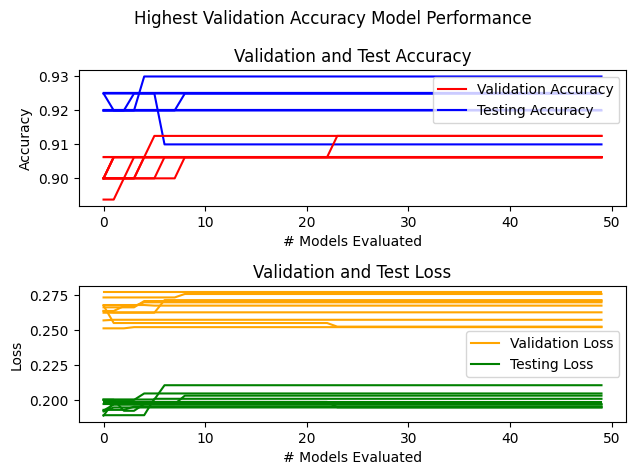# Conclusion

Hopefully these experiments provide insight into detecting overfitting, selecting validation split sizes, and deciding wether to use cross validation. Thanks for reading and feel free to contact me via email with any questions, comments, or feedback.